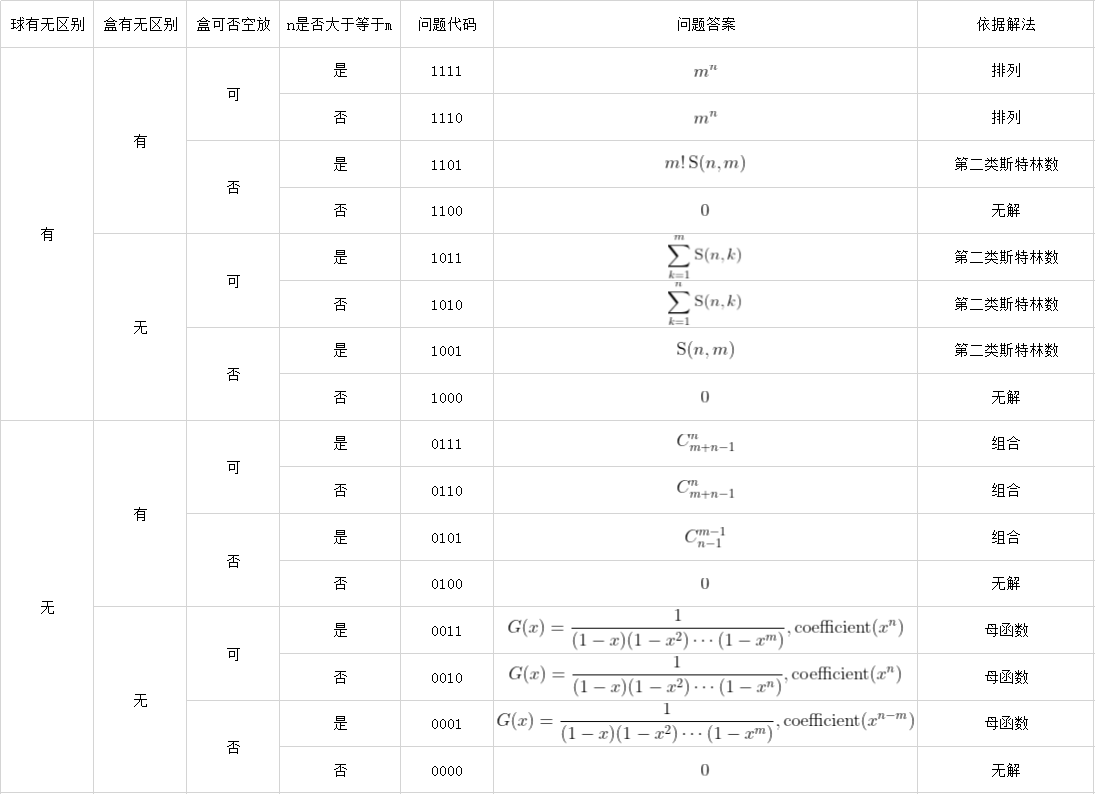# 前言

1. 球无区别，盒无区别，盒无空放，$n \ge m$
2. 球无区别，盒无区别，盒无空放，$n < m$
3. 球无区别，盒无区别，盒可空放，$n \ge m$
4. 球无区别，盒无区别，盒可空放，$n < m$
5. 球无区别，盒有区别，盒无空放，$n \ge m$
6. 球无区别，盒有区别，盒无空放，$n < m$
7. 球无区别，盒有区别，盒可空放，$n \ge m$
8. 球无区别，盒有区别，盒可空放，$n < m$
9. 球无区别，盒无区别，盒无空放，$n \ge m$
10. 球有区别，盒无区别，盒无空放，$n < m$
11. 球有区别，盒无区别，盒可空放，$n \ge m$
12. 球有区别，盒无区别，盒可空放，$n < m$
13. 球有区别，盒有区别，盒无空放，$n \ge m$
14. 球有区别，盒有区别，盒无空放，$n < m$
15. 球有区别，盒有区别，盒可空放，$n \ge m$
16. 球有区别，盒有区别，盒可空放，$n < m$

• 球有区别为1，球无区别为0
• 盒有区别为1，盒无区别为0
• 盒可空放为1，盒无空放为0
• $n \ge m$ 为1，$n < m$ 为0

{1, 2} {3} 和 {1, 3} {2} 是同一种情况

{1, 2} {3} 和 {3} {1, 2} 是同一种情况

{1, 3} {2}；{1, 2} {3}；{3} {1, 2}；{2} {1, 3} 全是一种情况。

1. 无解
2. 排列
3. 组合
4. 母函数
5. 第二类斯特林数

# 组合

## 0101

X X X X X X

X X|X X X X

X X|X|X X X

# 母函数

## 0011

$G(x) = (1 + x + x ^ 2 + \cdots)(1 + x ^ 2 + x ^ 4 + \cdots)\cdots(1 + x ^ m + x ^ {2m} + \cdots) = \dfrac{1}{(1 - x)(1 - x ^ 2)\cdots(1 - x ^ m)}$

## 0010

$G(x) = (1 + x + x ^ 2 + \cdots)(1 + x ^ 2 + x ^ 4 + \cdots)\cdots(1 + x ^ n + x ^ {2n} + \cdots) = \dfrac{1}{(1 - x)(1 - x ^ 2)\cdots(1 - x ^ n)}$

## 0001

$G(x) = (1 + x + x ^ 2 + \cdots)(1 + x ^ 2 + x ^ 4 + \cdots)\cdots(1 + x ^ m + x ^ {2m} + \cdots) = \dfrac{1}{(1 - x)(1 - x ^ 2)\cdots(1 - x ^ m)}$

# 第二类斯特林数

## 1001

### 容斥

$\operatorname{S}(n, m) = \dfrac{1}{m!}\sum^m_{k = 0}(-1)^kC^k_m(m - k) ^ n$

## 1011

$\sum ^ m _ {k = 1}\operatorname{S}(n, k)$

## 1010

$\sum ^ n _ {k = 1}\operatorname{S}(n, k)$

$\operatorname{B}(n) = \sum ^ n _ {k = 1}\operatorname{S}(n, k)$

# 总结# 参考资料

posted @ 2020-10-02 00:28  东北小蟹蟹  阅读(155)  评论(0编辑  收藏